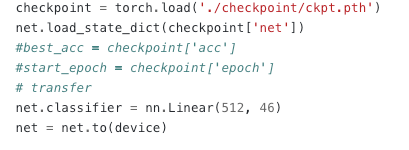# [Solved]Error when use 2 datasets to do transfer learning

Hi there. I trained a VGG network using CIFAR10 dataset first for transfer learning.
After I save the model of CIFAR 10 VGG network.
I substituted the classifier due to another dataset has different class number.Then I start to train the model with the new dataset.

``````def train(epoch):
print('\nEpoch: %d' % epoch)
net.train()
train_loss = 0
correct = 0
total = 0
for batch_idx, (inputs, targets) in enumerate(trainloader):
inputs, targets = inputs.to(device), targets.to(device)
outputs = net(inputs)
loss = criterion(outputs, targets)
loss.backward()
optimizer.step()

train_loss += loss.item()
_, predicted = outputs.max(1)
total += targets.size(0)
correct += predicted.eq(targets).sum().item()

progress_bar(batch_idx, len(trainloader), 'Loss: %.3f | Acc: %.3f%% (%d/%d)'
% (train_loss/(batch_idx+1), 100.*correct/total, correct, total))

train(net)
``````

However, there is an error.

I cannot get the point from the error message. Can you help me fix that? Many thanks.

Could you run the code on the CPU and check for error messages as they might be clearer?

I appreciate your help. Trying to run the code on CPU, I got a clear message.

The problem must be relevant to the changed class number between 2 datasets.

Yes, exactly. Check your targets to have values in the range `[0, nb_classes-1]`.

1 Like